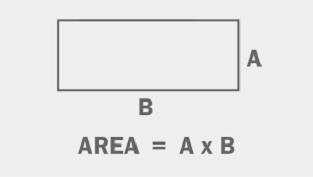# Haskell Program to Find the Area of a Rectangle

This tutorial discusses writing a program to print the area of a rectangle in the Haskell programming language.

The rectangle is a quadrilateral with opposite sides having the same length and adjacent sides at right angles. The area of a rectangle is equal to its product of length and breadth. If the length and breadth of the rectangle are 5 and 6 respectively, then the area of that is 30 units(5*6).In this tutorial, We see four ways to implement

• Program to compute the area of a rectangle using the infix operator “*”.

• Program to compute the area of a rectangle using the operator function “(*)”.

• Program to compute the area of a rectangle using the diagonal(when one among length or breadth is known).

• Program to compute the area of a rectangle using the Perimeter(when one among length or breadth is known)

## Algorithm Steps

• Take input or initialize the variable for the size of the rectangle.
• Implement the program logic to compute the area of the rectangle.
• Print or display the area.

## Example 1

Program to compute the area of a rectangle using the infix operator “*”

main ::IO()
main = do
-- initializing and declaring a variable for the length and breadth of the rectangle
let len = 5
let bred = 6
-- computing the area
let area = len*bred
-- printing the computed area
print ("Area of the rectangle with length "++ show len ++ "and breadth " ++ show bred ++" units is:")
print (area)


## Output

"Area of the rectangle with length 5 and breadth 6 units is:"
30


Note − The function show parses numbers into a string. It takes a number as an argument and returns the parsed string. “++” is an operator to concatenate strings.

In the above program, we declared and initialized variables to store the length and breadth of the rectangle. We computed the area of the rectangle by multiplying the length with breadth using the infix operator for multiplication(“*”) and loading the computed value into a variable area. Finally, we printed the area using the print function.

## Example 2

Program to compute the area of a rectangle using the operator function(“(*)”)

main ::IO()
main = do
-- initializing and declaring a variable for the length and breadth of the rectangle
let len = 5
let bred = 6
-- computing the area
let area =(*) len bred
-- printing the computed area
print ("Area of the rectangle with length "++ show len ++ " and breadth " ++ show bred ++" units is:")
print (area)


## Output

"Area of the rectangle with length 5 and breadth 6 units is:"
30


In the above program, we declared and initialized variables to store the length and breadth of the rectangle. We computed the area of the rectangle using the function operator for multiplication (“(*)”) by passing the length and breadth as arguments and loading the computed returned value into a variable area. Finally, we printed the area using the print function.

Note − Infix operators can be converted into operator functions by encapsulating them with round brackets. Then they can be used as general functions.

## Example 3

Program to compute the area of a rectangle using the diagonal(when one among length or breadth is known)

main ::IO()
main = do
-- initializing and declaring a variable for the length and breadth of the rectangle
let len = 5
let diagonal = 13
let breadth = sqrt (diagonal^2 - len^2)
-- computing the area
-- printing the computed area
print ("Area of the rectangle with length "++ show len ++ " and diagonal " ++ show diagonal ++" units is:")
print (area)


## Output

"Area of the rectangle with length 5.0 and diagonal 13.0 units is:"
60.0


In the above, we declared and initialized variables for length and diagonal of the rectangle. We computed the breadth of the rectangle from the length and diagonal using the Pythagoras principle. Computed the area by multiplying the length and breadth. Loaded the computed area into a variable area. Finally, printed the area of the rectangle.

## Example 4

Program to compute the area of a rectangle using the Perimeter(when one among length or breadth is known)

main ::IO()
main = do
-- initializing and declaring a variable for the length and breadth of the rectangle
let len = 5
let perimeter = 22
let breadth = (perimeter - len*2)/2
-- computing the area
-- printing the computed area
print ("Area of the rectangle with length "++ show len ++ " and perimeter " ++ show perimeter ++" units is:")
print (area)


## Output

"Area of the rectangle with length 5.0 and perimeter 22.0 units is:"
30.0


Note − In a rectangle opposite sides are of the same length, i.e 2*length and 2*breadth is equal to the perimeter of the rectangle. We can compute the length or breadth if we know the perimeter and one of the length or breadth.

In the above program. We declared and initialized variables for the length and perimeter of the rectangle. We computed the breadth of the rectangle from the perimeter and length. Computed the area by multiplying the length and breadth. Loaded the computed area into a variable area. Finally, printed the area of the rectangle.

## Conclusion

In this tutorial, we discussed four ways to implement the program to compute the area of the rectangle in the Haskell programming language.